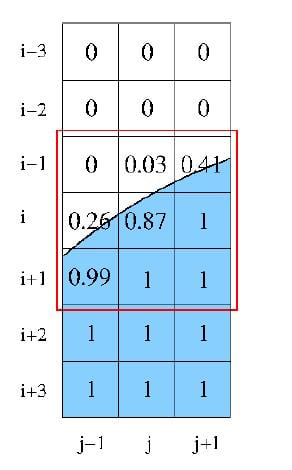# Calculating the curvature of an interface in VOF model

Does anyone have any idea about how to calculate the curvature of an interface between two phases while using the VOF model? There are a few algorithms out there but they are not very clear. For example, the young's algorithm, the height function algorithm. In which we have to calculate Delta(x) and Delta(y) and access the volume fractions of nearby cells. I have trouble in doing both.

Can you help me calculating the Delta (x) and Delta(y) (basically the grid spacing) and how to use the C_VOF of nearby cells?

Thanks

Gunjan

• edited February 2019

Check the Continuum Surface Force Model in Theory Guide and you have your formula.

• edited February 2019

Thanks about that! But I don't want an algorithm, I need to know how to implement it. Can you help me calculating the Delta (x) and Delta(y) (basically the grid spacing) and how to use the C_VOF of neighboring cells?

Thanks,

Gunjan

• edited February 2019

Curvature is the laplacian of the volume fraction: So you need to get the gradients and then build up the divergence by bulding up the gradient of the gradient and then just summing up what one requires to get the laplacian.

• edited February 2019

Yeah I know that, but how would you calculate gradient of that gradient again? Do I need to store the gradient of volume fraction in another UDS and then use C_UDSI_G? because to calculate the gradient in a c code, we will need to discretize the equation. For which we'll need to access the mesh elements one by one like in the figure. That's what I'm asking how can I access a cell at (i,j)?• edited February 2019

You can work that with User Defined Scalars: You create them you save the VOG_G in them and then you get the Gradients out of them: Do not solve the equations for the scalar.